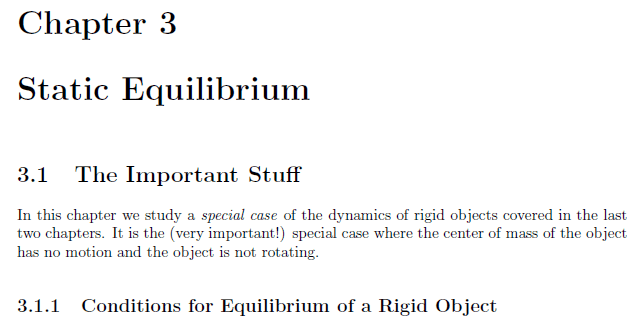# The Force Of The Ladder Against The Wall

Coldry
OK folks, I'm sure this will be easy for the brains in this forum, but I've always been curious as to how this is done. So if you have situation where you have a ladder leaning on a wall and a person is some way up the ladder. If you know the length of the ladder (3m), the angle the ladder is leaning (50 Degrees at the bottom inside the triangle made by the ladder the floor and the wall), the weight of the ladder (10 kg), the weight of the person (110kg) and exactly how high up the ladder the person is (2.5m), how would you calculate the force the ladder is applying on the wall at the top of the ladder?

So I've put some random numbers for parameters here. I don't understand or know the maths involved, so if you need to make some of these numbers different to simplify the explanation please go ahead. Drawings would be appreciated.

Thanks

Homework Helper
Hello @Coldry,##\qquad## !​

I can understand you feel unable to do the calculation, but I have little patience with
Drawings would be appreciated.
because I have absolutely no idea why you can't come up with at least an initial sketch !

So post that (*) and then we'll talk about the necessary equilibrium of forces, as well as about the equilibrium of torques that is needed to solve this one.
By the way, is this homework? Because in PF, this exercise definitely qualifies as such...

 (*) that will also show how realistic your random parameter values appear##\ ##

Mentor
I don't understand or know the maths involved,
Have a look at this introductory reference to see if it helps: